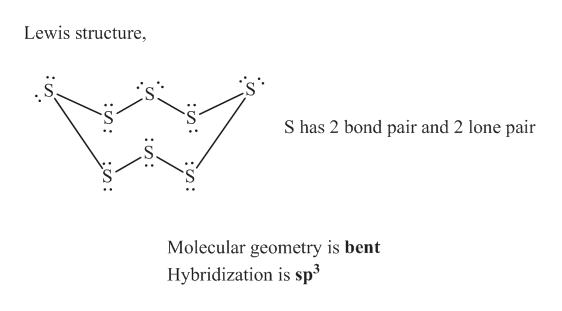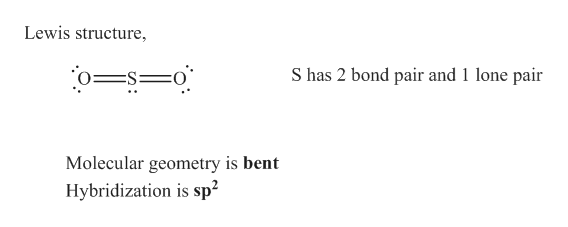# Sulfuric acid is manufactured by a series of reactions represented by the following equations:S8(s)+8O2(g) ⟶ 8SO2(g)2SO2(g)+O2(g) ⟶ 2SO3(g)SO3(g)+H2O(l) ⟶ H2SO4(l)Draw a Lewis structure, predict the molecular geometry by VSEPR, and determine the hybridization of sulfur for the following:(a) circular S8 molecule(b) SO2 molecule(c) SO3 molecule(d) H2SO4 molecule (the hydrogen atoms are bonded to oxygen atoms)

Question

Sulfuric acid is manufactured by a series of reactions represented by the following equations:

S8(s)+8O2(g) ⟶ 8SO2(g)

2SO2(g)+O2(g) ⟶ 2SO3(g)

SO3(g)+H2O(l) ⟶ H2SO4(l)

Draw a Lewis structure, predict the molecular geometry by VSEPR, and determine the hybridization of sulfur for the following:

(a) circular S8 molecule

(b) SO2 molecule

(c) SO3 molecule

(d) H2SO4 molecule (the hydrogen atoms are bonded to oxygen atoms)

check_circleExpert Solution
Step 1

The Lewis electron dot structure for given molecules are determined by first drawing the skeletal structure for the given molecules, then the total number of valence electrons for all atoms present in the molecules are determined.

The next step is to subtract the electrons present in the total number of bonds present in the skeletal structure of the molecule with the total valence electrons such that considering each bond contains two electrons with it.

Finally, the electrons which got after subtractions have to be equally distributed considering each atom contains eight electrons in its valence shell.

Step 2

(a) Circular S8 molecule

In VSEPR, the geometry of the molecule is explained based on minimizing electrostatic repulsion between the molecules’ valence electrons around a central atom.

Hybridization is the mixing of valence atomic orbitals to get equivalent hybridized orbitals that having similar characteristics and energy.

Lewis structure the molecular geometry by VSEPR, and the hybridization of sulfur of circular S8 molecule is given below,

Outer valence electrons of Sulfur are six.help_outlineImage TranscriptioncloseLewis structure S has 2 bond pair and 2 lone pair Molecular geometry is bent Hybridization is sp3 fullscreen
Step 3

(b)

Lewis structure the molecular geometry by VSEPR, and the hybridization of sulfur of SO2 molec...help_outlineImage TranscriptioncloseLewis structure S has 2 bond pair and 1 lone pair Molecular geometry is bent Hybridization is sp2 fullscreen

### Want to see the full answer?

See Solution

#### Want to see this answer and more?

Solutions are written by subject experts who are available 24/7. Questions are typically answered within 1 hour*

See Solution
*Response times may vary by subject and question
Tagged in

### Physical Chemistry